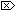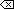The Open Group Base Specifications Issue 6
IEEE Std 1003.1-2001

#### NAME

log1p, log1pf, log1pl - compute a natural logarithm

#### SYNOPSIS

```#include <math.h> double log1p(double x); float log1pf(float x); long double log1pl(long double x); ```

#### DESCRIPTION

[CX]The functionality described on this reference page is aligned with the ISO C standard. Any conflict between the requirements described here and the ISO C standard is unintentional. This volume of IEEE Std 1003.1-2001 defers to the ISO C standard.These functions shall compute loge(1.0 + x).

An application wishing to check for error situations should set errno to zero and call feclearexcept(FE_ALL_EXCEPT) before calling these functions. On return, if errno is non-zero or fetestexcept(FE_INVALID | FE_DIVBYZERO | FE_OVERFLOW | FE_UNDERFLOW) is non-zero, an error has occurred.

#### RETURN VALUE

Upon successful completion, these functions shall return the natural logarithm of 1.0 + x.

If x is -1, a pole error shall occur and log1p(), log1pf(), and log1pl() shall return -HUGE_VAL, -HUGE_VALF, and -HUGE_VALL, respectively.

For finite values of x that are less than -1, [MX]or if x is -Inf,a domain error shall occur, and [MX]either a NaN (if supported), oran implementation-defined value shall be returned.

[MX]If x is NaN, a NaN shall be returned.

If x is ±0, or +Inf, x shall be returned.

If x is subnormal, a range error may occur and x should be returned.#### ERRORS

These functions shall fail if:

Domain Error
The finite value of x is less than -1, [MX]or x is -Inf.If the integer expression (math_errhandling & MATH_ERRNO) is non-zero, then errno shall be set to [EDOM]. If the integer expression (math_errhandling & MATH_ERREXCEPT) is non-zero, then the invalid floating-point exception shall be raised.

Pole Error
The value of x is -1.

If the integer expression (math_errhandling & MATH_ERRNO) is non-zero, then errno shall be set to [ERANGE]. If the integer expression (math_errhandling & MATH_ERREXCEPT) is non-zero, then the divide-by-zero floating-point exception shall be raised.

These functions may fail if:

Range Error
[MX]The value of x is subnormal.

If the integer expression (math_errhandling & MATH_ERRNO) is non-zero, then errno shall be set to [ERANGE]. If the integer expression (math_errhandling & MATH_ERREXCEPT) is non-zero, then the underflow floating-point exception shall be raised.The following sections are informative.

None.

#### APPLICATION USAGE

On error, the expressions (math_errhandling & MATH_ERRNO) and (math_errhandling & MATH_ERREXCEPT) are independent of each other, but at least one of them must be non-zero.

None.

#### FUTURE DIRECTIONS

None.

feclearexcept() , fetestexcept() , log() , the Base Definitions volume of IEEE Std 1003.1-2001, Section 4.18, Treatment of Error Conditions for Mathematical Functions, <math.h>

#### CHANGE HISTORY

First released in Issue 4, Version 2.

#### Issue 5

Moved from X/OPEN UNIX extension to BASE.

#### Issue 6

The DESCRIPTION is updated to avoid use of the term "must" for application requirements.

The log1p() function is no longer marked as an extension.

The log1pf() and log1pl() functions are added for alignment with the ISO/IEC 9899:1999 standard.

The DESCRIPTION, RETURN VALUE, ERRORS, and APPLICATION USAGE sections are revised to align with the ISO/IEC 9899:1999 standard.

IEC 60559:1989 standard floating-point extensions over the ISO/IEC 9899:1999 standard are marked.

End of informative text.

UNIX ® is a registered Trademark of The Open Group.
POSIX ® is a registered Trademark of The IEEE.
[ Main Index | XBD | XCU | XSH | XRAT ]